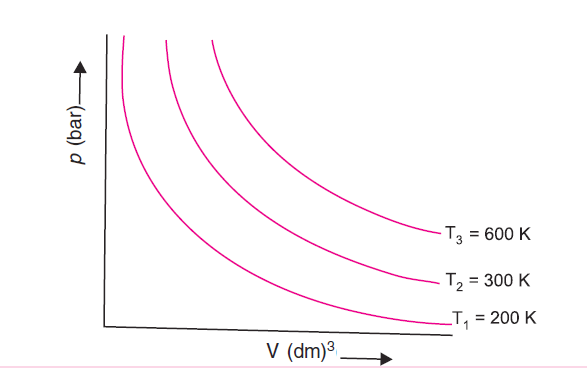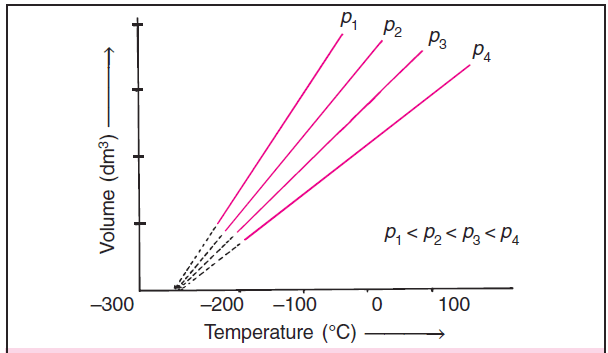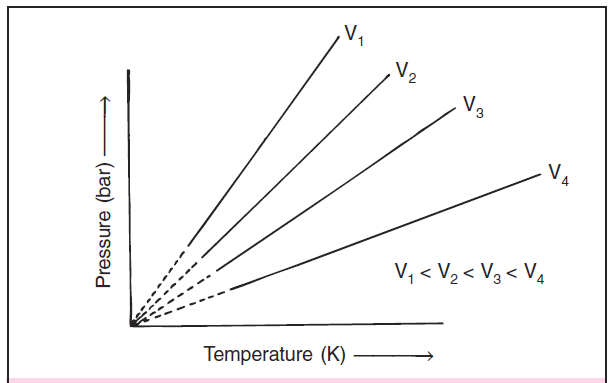# Isotherm, Isobar & Isochore graphs

When we study gas laws (Boyle’s law, Charles’ law & Pressure Law), we come across terms like Isotherm, Isobar, and Isochore. Here, in this post, we will discuss these.

## What is Isotherm?

If we draw Boyle’s law graphs at diff temperatures then we get curves shown in figure 1.figure 1: Boyle’s law graphs at different temperatures. Each curve corresponds to a different constant temperature and is known as an isotherm.

Boyle’s law is expressed as V = k1 / p where V is the volume, p is the pressure of the gas and k1 is a constant of proportionality whose value depends upon the amount of the gas and temperature.

The value of k1 for each curve is different (because for a given mass of gas it varies only with temp).

Each p-V curve of Boyle’s law graphs corresponds to a different constant temperature and is known as an isotherm. Higher curves correspond to higher temp.

## What is Isobar?

If we draw Charles’ law graphs at different pressures then we get curves shown in figure 2.figure 2: Charles’ law graphs at different pressures. Each curve corresponds to different constant pressure and is known as an isobar.

Charles’ law is expressed as V = k2 T where V is the volume, T is the temperature of the gas and k2 is a constant of proportionality.

These Charles’ law curves are Volume vs temperature (°C) graphs for different pressures.

Each line of the Charles’ law graphs or Volume vs Temperature graphs corresponds to a different constant pressure and is called isobar.

## What is Isochore?

If we draw Pressure law graphs at different volumes then we get curves shown in figure 3.figure 3: Pressure law graphs at different volumes. Each curve corresponds to a different constant volume and is known as an isochore.

Pressure law is expressed as P = k3 T where P is the pressure, T is the temperature of the gas and k3 is a constant of proportionality.

These Pressure law curves are Pressure vs temperature (°C) graphs for different volumes.

Each line of the Pressure law graphs or Pressure vs Temperature graphs corresponds to a different constant volume and is called isochore.